# Magnetic Field Formula

Magnetic Field Formula

When electric current is carried in a wire, a magnetic field is formed around it. The magnetic field lines form concentric circles around the wire. The magnetic field direction depends on the direction of the current. It can be determined using the "right hand rule", by pointing the thumb of your right hand in the direction of the current. The direction of the magnetic field lines is the direction of your curled fingers. The magnitude of the magnetic field depends on the amount of current, and the distance from the charge-carrying wire. The formula includes the constant. This is called the permeability of free space, and has a value. The unit of magnetic field is the Tesla, T.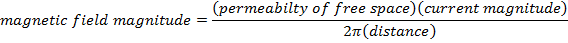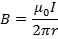B = magnetic field magnitude (Tesla, T)= permeability of free space ()

I = magnitude of the electric current (Amperes, A)

r = distance (m)

Magnetic Field Formula Questions:

1) What is the magnitude of the magnetic field 0.10 m away from a wire carrying a 3.00 A current? If the current has a vector direction out of the page (or screen), what is the direction of the magnetic field?

Answer: The magnitude of the magnetic field can be calculated using the formula: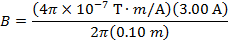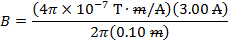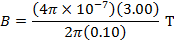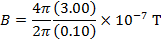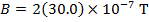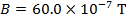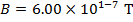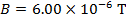The magnitude of the magnetic field is 6.00 x 10-6 T, which can also be written as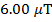(micro-Tesla).

The direction of the magnetic field can be determined using the "right hand rule", by pointing the thumb of your right hand in the direction of the current. The direction of the magnetic field lines is the direction of your curled fingers. The current has a vector direction out of the page, and so your fingers will curl in the counter-clockwise direction. Therefore, the magnetic field lines point in the counter-clockwise direction, forming circles around the wire.

2) If the magnitude of a magnetic field 2.00 m away from a wire is 10.0 nT(nano-Tesla), what is the magnitude of the electric current carried by the wire? If the magnetic field lines form clockwise circles in the plane of the page (or screen), what is the vector direction of the electric current?

Answer: The magnitude of the electric current can be calculated by rearranging the magnetic field formula: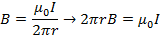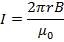The magnitude of the magnetic field is given in nano-Tesla. The prefix "nano" means 10-9, and so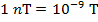. The magnitude of the magnetic field at the distance specified is thus:

B = 10.0 nT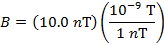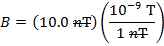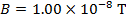The magnitude of the current in the wire is: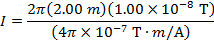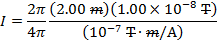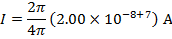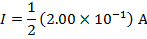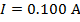The magnitude of the electric current in the wire is 0.100A.

The direction of the electric current can be determined using the "right hand rule". The magnetic field lines form clockwise circles in the plane of the page, so imagine curling your right hand so that your fingers point in the clockwise direction. To do this, your thumb must point toward the page (or screen). Therefore, the direction of the electric current is into the page (or screen).